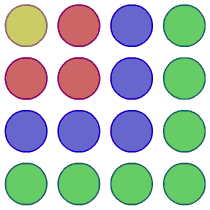### Submit a Resource

NRICH: Picturing Square Numbers

Square numbers can be represented as the sum of consecutive odd numbers. What is the sum of 1 + 3 + ….. + 149 + 151 + 153?

The diagram shows that 1 + 3 + 5 + 7 = 16.What is the sum of the first 30 odd numbers?

What is the sum of the first 60 odd numbers?

Can you describe a method for working these out quickly?

Can you make 3249 by adding odd numbers in this way?

What is the value of:

1+3+...+149+151+153?

83+81+...+5+3+1?

51+53+55+...+149+151+153?

2+4+...+150+152+154?

2+6+...+298+302+306?

Explain how you worked these out.

Age 11 to 14

Math Topics
Algebra & Pre-Algebra, Patterning & Sequencing
Middle School, High School, Educator

## Organization

NRICH (University of Cambridge)

## Type of Resource

Challenge
Online Interactive Activity